MCAT Physical : Work

Example Questions

2 Next →

Example Question #11 : Work, Energy, And Power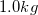block slides along a smooth surface at a velocity of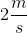. The block then encounters a rough surface and continues for six meters before coming to a complete stop. What is the force of friction on the block?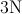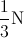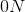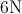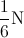Explanation:

This question can be answered using conservation of energy. Having an understanding of the work-energy theorem allows us the knowledge that changing kinetic energy is a form of work, and that work can be a form of energy. In this particular question, the only force acting on the block to slow it down is the force of friction from the rough surface. As we know, work is equal to force times distance.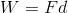Because the block started with a certain amount of kinetic energy, and then is brought to a complete stop, all of that kinetic energy is transferred to work done by the force of friction over a distance of six meters.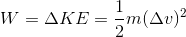Because theblock had a velocity ofon a smooth surface and ends with a velocity of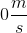at rest, we can calculate the change in kinetic energy.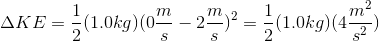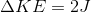The kinetic energy is completely converted to the work done by friction.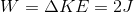We can then use the first equation for work to determine the force of friction.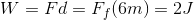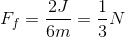Example Question #11 : Work

A 2kg box slides down a ramp a distance of two meters before it reaches the ground. The ramp has an angle of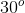. The coefficient of kinetic friction for the ramp is 0.1.

What is the work done on the box by friction?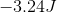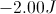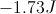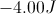Explanation:

Because the force of friction is parallel to the displacement of the box, we can solve for the work done by friction using the following equation.Now, we need to define the force of friction or.In this formula,equals acceleration. In this case, acceleration equals acceleration due to gravity in the x-direction; therefore, use the cosine function.Insert the value of acceleration due to gravity for.Now, we can solve for the work done by friction.2 Next →